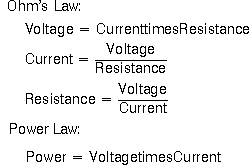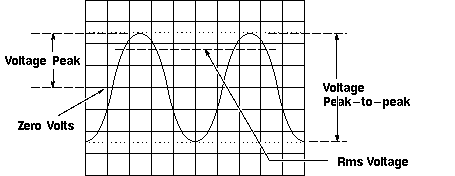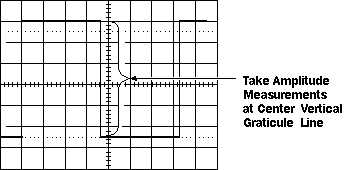Home > Electronic Tutorials > Oscilloscope Tutorial > Oscilloscope Voltage Measurements

Online Oscilloscope Tutorial

# About OSCILLOSCOPE Oscilloscope Voltage Measurements

## Voltage Measurements in Oscilloscope

Voltage is the amount of electric potential, expressed in volts, between two points in a circuit. Usually one of these points is ground (zero volts) but not always. Voltages can also be measured from peak-to-peak - from the maximum point of a signal to its minimum point. You must be careful to specify which voltage you mean.

The oscilloscope is primarily a voltage-measuring device. Once you have measured the voltage, other quantities are just a calculation away. For example, Ohm's law states that voltage between two points in a circuit equals the current times the resistance. From any two of these quantities you can calculate the third. Another handy formula is the power law: the power of a DC signal equals the voltage times the current. Calculations are more complicated for AC signals, but the point here is that measuring the voltage is the first step towards calculating other quantities.Following figure shows the voltage of one peak - V[p] - and the peak-to-peak voltage - V[p-p] -, which is usually twice V[p]. Use the RMS (root-mean-square) voltage - V[RMS] - to calculate the power of an AC signal.Voltage Peak and Peak-to-peak Voltage

You take voltage measurements by counting the number of divisions a waveform spans on the oscilloscope's vertical scale. Adjusting the signal to cover most of the screen vertically, then taking the measurement along the center vertical graticule line having the smaller divisions, makes for the best voltage measurements. The more screen area you use, the more accurately you can read from the screen.Measure Voltage on the Center Vertical Graticule Line

Many oscilloscopes have on-screen cursors that let you take waveform measurements automatically on-screen, without having to count graticule marks. Basically, cursors are two horizontal lines for voltage measurements and two vertical lines for time measurements that you can move around the screen. A readout shows the voltage or time at their positions.

Note: To report broken links or to submit your projects please send email to Webmaster

 Discover